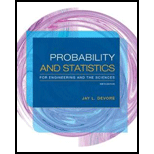# A computer consulting firm presently has bids out on three projects. Let A i = {awarded project i }, for i = 1, 2, 3, and suppose that P ( A 1 ) = .22, P ( A 2 ) = .25, P ( A 3 ) = .28, P ( A 1 ∩ A 2 ) = .11, P ( A 1 ∩ A 3 ) = .05, P ( A 2 ∩ A 3 ) = .07, P ( A 1 ∩ A 2 ∩ A 3 ) = .01. Express in words each of the following events, and compute the probability of each event: a. A 1 ∪ A 2 b. A 1 ′∩ A 2 ′ [ Hint: ( A 1 ∪ A 2 )′ = A ′ 1 ∩ A ′ 2 ] c. A 1 ∪ A 2 ∪ A 3 d. A ′ 1 ∩ A ′ 2 ∩ A ′ 3 e. A ′ 1 ∩ A ′ 2 ∩ A 3 f. ( A ′ 1 ∩ A ′ 2 ) ∪ A 3### Probability and Statistics for Eng...

9th Edition
Jay L. Devore
Publisher: Cengage Learning
ISBN: 9781305251809### Probability and Statistics for Eng...

9th Edition
Jay L. Devore
Publisher: Cengage Learning
ISBN: 9781305251809

#### Solutions

Chapter
Section
Chapter 2.2, Problem 13E
Textbook Problem

## Expert Solution

### Want to see the full answer?

Check out a sample textbook solution.See solution

### Want to see this answer and more?

Experts are waiting 24/7 to provide step-by-step solutions in as fast as 30 minutes!*

See Solution

*Response times vary by subject and question complexity. Median response time is 34 minutes and may be longer for new subjects.